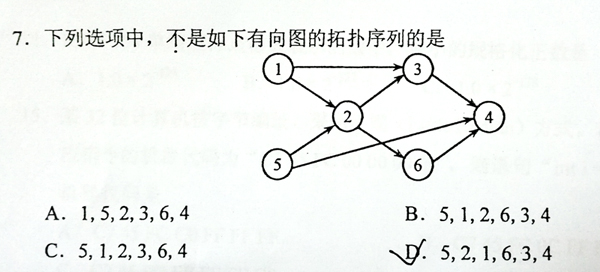# PAT-A 真题 – 1146 Topological Order

This is a problem given in the Graduate Entrance Exam in 2018: Which of the following is NOT a topological order obtained from the given directed graph? Now you are supposed to write a program to test each of the options.### Input Specification:

Each input file contains one test case. For each case, the first line gives two positive integers N ( 1,000), the number of vertices in the graph, and M ( 10,000), the number of directed edges. Then M lines follow, each gives the start and the end vertices of an edge. The vertices are numbered from 1 to N. After the graph, there is another positive integer K ( 100). Then K lines of query follow, each gives a permutation of all the vertices. All the numbers in a line are separated by a space.

### Output Specification:

Print in a line all the indices of queries which correspond to "NOT a topological order". The indices start from zero. All the numbers are separated by a space, and there must no extra space at the beginning or the end of the line. It is graranteed that there is at least one answer.

### Sample Input:

6 8
1 2
1 3
5 2
5 4
2 3
2 6
3 4
6 4
5
1 5 2 3 6 4
5 1 2 6 3 4
5 1 2 3 6 4
5 2 1 6 3 4
1 2 3 4 5 6

### Sample Output:

3 4

#include <iostream>
#include <bits/stdc++.h>
using namespace std;

int main(){
int pcnt, vcnt;
cin >> pcnt >> vcnt;
vector<int> inv(pcnt + 1, 0);
vector<vector<int> > G(pcnt + 1);
for(int i = 0; i < vcnt; i++){
int l, r;
cin >> l >> r;
inv[r]++;
G[l].push_back(r);
}
int qcnt;
cin >> qcnt;
vector<int> ans;
for(int i = 0; i < qcnt; i++){
vector<int> tmpinv = inv;
int flag = false;
for(int i = 0; i < pcnt; i++){
int n;
cin >> n;
if(tmpinv[n] != 0) flag = true;
if(flag == false)
for(int it : G[n]) tmpinv[it]--;
}
if(flag) ans.push_back(i);
}
for(int i = 0; i < ans.size(); i++){
if(i) cout << ' ';
cout << ans[i];
}
cout << endl;
return 0;
}